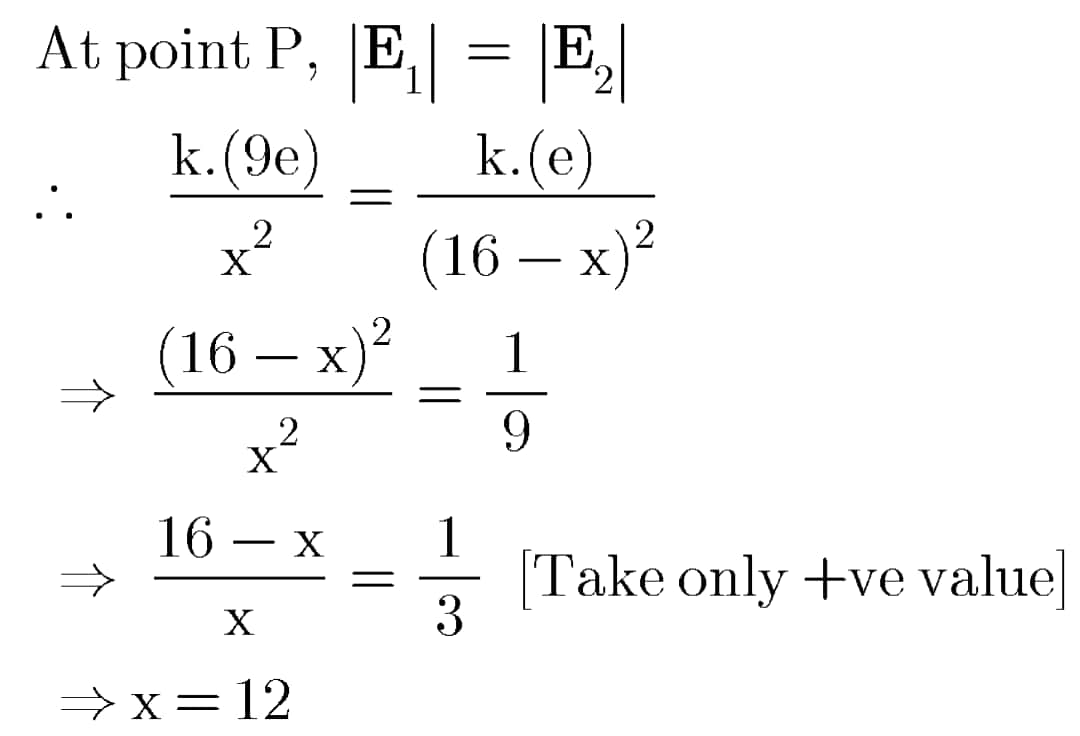# Electric Field and Potential: Problem 1.01, 1.02 & 1.03 with detailed Solutions

### 1.01) Taking mass (M), length (L), time (T) and current (I) as base quantities, the dimensional formula of permittivity is :A) ML⁻²T²I B) M⁻¹L⁻³T⁴I² C) MLT⁻²I D) ML²T⁻¹I²

The magnitude of Coulomb Force between two point charges q₁ and q₂ is: F = |q₁q₂|/4πεᤱr² where εᤱ is the permittivity of vaccum. From this expression of force, we have εᤱ = |q₁q₂|/4πr²F. Therefore,
Hence the dimensions formula of permittivity is [M⁻¹L⁻³T⁴I²].

### 1.02) Two point charges +9e and +e are placed 16 cm apart from each other. Where should a third point charge q be positioned between them so that the system of charges is in electrostatic equilibrium state? A) 24 cm from +9e B) 12 cm from +9e C) 24 cm from +e D) 12 cm from +e The third point charge q should be placed at a point where the net electric field produced by the system of remaining two point charges (+9e and +e) is zero. Only then, the charge q will be in equilibrium. Also if we choose the appropriate value of the charge q (which we can calculate, but not needed here to answer the problem), the whole system of the three point charges will be in the state of electrostatic equilibrium. Let P be a point where the resultant field due to the point charges +9e and +e is zero. It means that, at point P, the individual fields produced by +9e and +e are equal and oppositely directed, hence cancelling each other's effect. By inspection, it is conclusive that point P is unique and it lies on the line joining the two point charges and in between the two charges. Also, it will lie closer to the smaller magnitude charge. Let the point P is located at a distance x from the charge +9e. Or, it's distance from the charge +e is (16 cm - x). Since the resultant electric field vector at P is zero, we can write using Coulomb's law,Hence the third charge q should be placed at a point P which is located on the line joining the rest two charges and at a distance of 12 cm from the charge +9e. 1.03) When electric charge is provided to a soap bubble, it shows : A) an increase in size B) sometimes an increase and sometimes a decrease C) no change in size D) none of these

When we provide some charge to a soap bubble, the bubble gets either a net positive charge or net negative charge. The likely charged particles will get evenly distributed over the surface of the bubble and will repel each other due to the electrostatic repulsion. Hence, size of the bubble will tend to increase.

Hindi Hearts